##1. Hyperbola with respect to its asymptotics

The figure shows how to determine the axes of the hyperbola, knowing its equation xy=c˛/4, with respect to its asymptotic lines OX and OY (X, Y control-points): Draw the circle with radius c=|OF|, find ABCD, F, F* and E. Look in the file HyperbolaAsymptotics.html for the equation of the hyperbola with respect to its principal axes. c is controlled by a number object. In the figure we calculate the coordinates (x,y) of a variable point Z of the hyperbola with respect to the asymptotics. The calculation verifies that xy = c˛/4.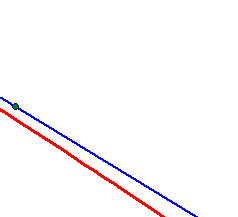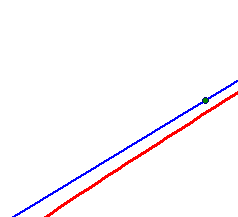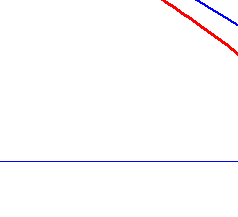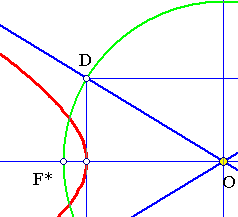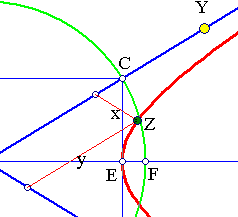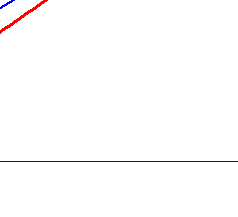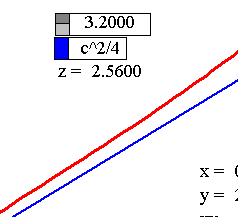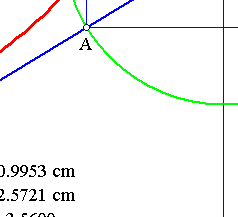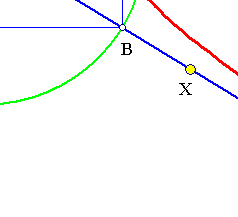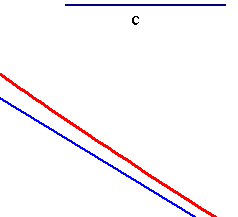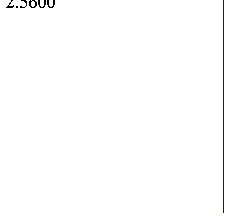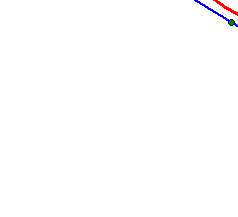Look at HyperbolaProperty.html for a nice application of this to the determination of related geometric locus.

##2. A family of similar hyperbolas

The family of conics generated by the hyperbola and its asymptotes can be described through the equation (xy-c2/4) + k(xy) = 0, equivalent to (xy)(1+k)-c2/4=0. This shows that the whole family consists of conics having the same asymptotes and being similar to each other.